cancel
Showing results for
Did you mean:New Member

## Calculation based on previous row with dependency between columns

I have some simple excel formulas that I would like to calculate in power query M. The issue I see is that each row is dependent on the outcome of the previous row but that there is also a column dependency as well

• total = previous row total + fixed value + loss
• loss = previous row total * multiplier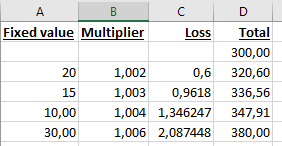I have already looked at index columns, List.Sum function, etc but I don't get what I'm looking for because of the column dependency.
How can I make this work in power query?

1 ACCEPTED SOLUTIONCommunity Support

Hi @Fram ,

Sorry for my wrong understanding.

If your Loss is calculated in terms of Total, but your Total is also calculated in terms of Loss, it seems impossible to implement in Power Query.

This is because in Excel, each cell of yours can be treated as an independent operation, not in Power Query.

Best Regards,

Stephen Tao

If this post helps, then please consider Accept it as the solution to help the other members find it more quickly.

3 REPLIES 3Community Support

Hi @Fram ,

Here's my solution.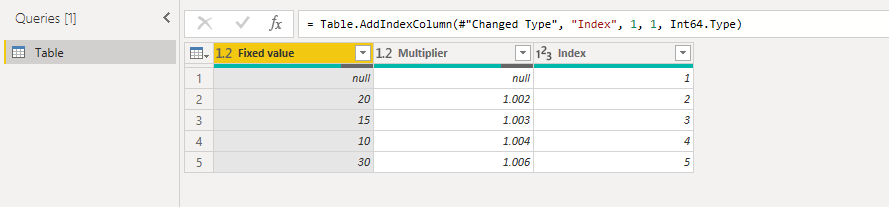2.Add a custom column as Total.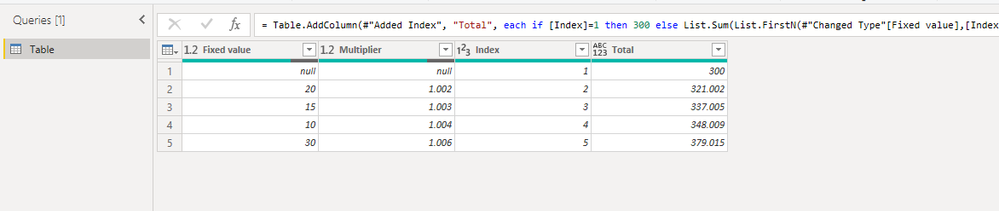3.Get the previous row based on Total column. Reference: Value from previous row – Power Query, M language – Trainings, consultancy, tutorials (exceltown.com...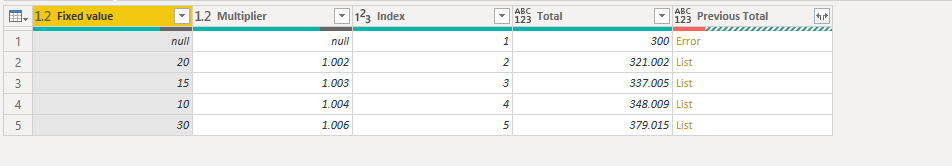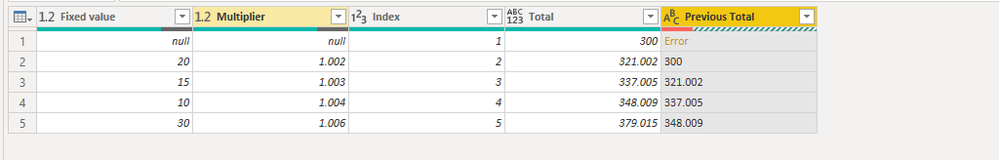Replace error with blank.4.After change the type Previous Total to Decimal Number. We can add a custom column to get Loss.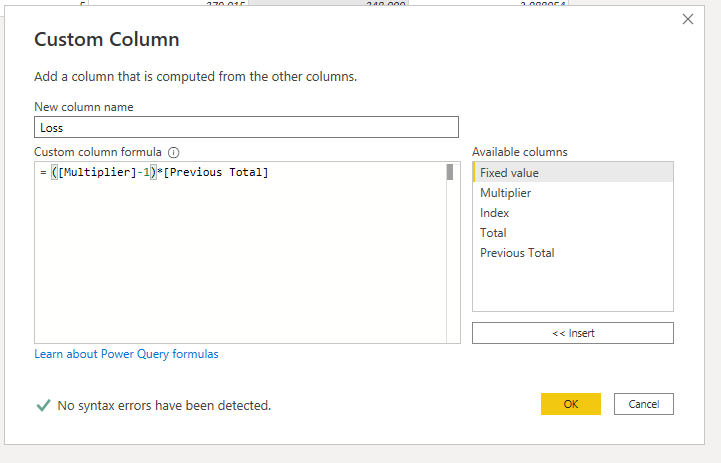Remove the unneeded columns.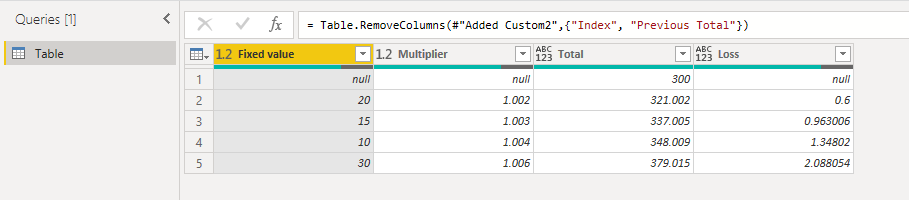Best Regards,

Stephen Tao

If this post helps, then please consider Accept it as the solution to help the other members find it more quickly.New Member

Hi,

Thanks for your contribution. If you compare the values you will see that the result is different, so no solution for me so far.
The issue I see is in step 2: you calculate a custom total, but this one does not include the loss column and it should (for some reason you add the multiplier to the fixed value, while in fact the multiplier in the excel formulae applies to the previous total, with the issue that the previous total again is dependend on the loss of the previous total and the previous previous total).Community Support

Hi @Fram ,

Sorry for my wrong understanding.

If your Loss is calculated in terms of Total, but your Total is also calculated in terms of Loss, it seems impossible to implement in Power Query.

This is because in Excel, each cell of yours can be treated as an independent operation, not in Power Query.

Best Regards,

Stephen Tao

If this post helps, then please consider Accept it as the solution to help the other members find it more quickly.